# 5   Determining combined standard uncertainty

## 5.1   Uncorrelated input quantities

This subclause treats the case where all input quantities are independent (C.3.7). The case where two or more input quantities are related, that is, are interdependent or correlated (C.2.8), is discussed in 5.2.

5.1.1   The standard uncertainty of y, where y is the estimate of the measurand Y and thus the result of the measurement, is obtained by appropriately combining the standard uncertainties of the input estimates x1x2, ..., xN (see 4.1). This combined standard uncertainty of the estimate y is denoted by uc(y).

NOTE   For reasons similar to those given in the note to 4.3.1, the symbols uc(y) and u2c(y) are used in all cases.

5.1.2   The combined standard uncertainty uc(y) is the positive square root of the combined variance u2c(y), which is given by(10)
where f is the function given in Equation (1). Each u(xi) is a standard uncertainty evaluated as described in 4.2 (Type A evaluation) or as in 4.3 (Type B evaluation). The combined standard uncertainty uc(y) is an estimated standard deviation and characterizes the dispersion of the values that could reasonably be attributed to the measurand Y (see 2.2.3).

Equation (10) and its counterpart for correlated input quantities, Equation (13), both of which are based on a first‑order Taylor series approximation of Y = f(X1X2, ..., XN), express what is termed in this Guide the law of propagation of uncertainty (see E.3.1 and E.3.2).

NOTE   When the nonlinearity of f is significant, higher‑order terms in the Taylor series expansion must be included in the expression for u2c(y), Equation (10). When the distribution of each Xi is normal, the most important terms of next highest order to be added to the terms of Equation (10) areSee H.1 for an example of a situation where the contribution of higher-order terms to u2c(y) needs to be considered.

5.1.3   The partial derivatives f xi are equal to f Xi evaluated at Xi = xi (see Note 1 below). These derivatives, often called sensitivity coefficients, describe how the output estimate y varies with changes in the values of the input estimates x1x2, ..., xN. In particular, the change in y produced by a small change Δxi in input estimate xi is given by y)i = (fxi)(Δxi). If this change is generated by the standard uncertainty of the estimate xi, the corresponding variation in y is (f xi)u(xi). The combined variance u2c(y) can therefore be viewed as a sum of terms, each of which represents the estimated variance associated with the output estimate y generated by the estimated variance associated with each input estimate xi. This suggests writing Equation (10) as(11a)
where(11b)

NOTE 1   Strictly speaking, the partial derivatives are fxi = fXi evaluated at the expectations of the Xi. However, in practice, the partial derivatives are estimated by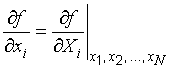NOTE 2   The combined standard uncertainty uc(y) may be calculated numerically by replacing ciu(xi) in Equation (11a) withThat is, ui(y) is evaluated numerically by calculating the change in y due to a change in xi of +u(xi) and of u(xi). The value of ui(y) may then be taken as Zi and the value of the corresponding sensitivity coefficient ci as Ziu(xi).

EXAMPLE   For the example of 4.1.1, using the same symbol for both the quantity and its estimate for simplicity of notation,and5.1.4   Instead of being calculated from the function f, sensitivity coefficients f xi are sometimes determined experimentally: one measures the change in Y produced by a change in a particular Xi while holding the remaining input quantities constant. In this case, the knowledge of the function f (or a portion of it when only several sensitivity coefficients are so determined) is accordingly reduced to an empirical first‑order Taylor series expansion based on the measured sensitivity coefficients.

5.1.5   If Equation (1) for the measurand Y is expanded about nominal values Xi,0 of the input quantities Xi, then, to first order (which is usually an adequate approximation), Y = Y0 + c1δ1 + c2δ2 + ... + cNδN, where Y0 = f(X1,0X2,0, ..., XN,0), ci = (fXi) evaluated at Xi = Xi,0, and δi = Xi − Xi,0. Thus, for the purposes of an analysis of uncertainty, a measurand is usually approximated by a linear function of its variables by transforming its input quantities from Xi to δi (see E.3.1).

EXAMPLE   From Example 2 of 4.3.7, the estimate of the value of the measurand V is V = V‾‾ + ΔV‾‾, where V‾‾ = 0,928 571 V, u(V‾‾) = 12 µV, the additive correction ΔV‾‾ = 0, and uV‾‾) = 8,7 µV. Since V V‾‾ = 1, and V ∂(ΔV‾‾) = 1, the combined variance associated with V is given byand the combined standard uncertainty is uc(V) = 15 µV, which corresponds to a relative combined standard uncertainty uc(V) V of 16 × 10−6 (see 5.1.6). This is an example of the case where the measurand is already a linear function of the quantities on which it depends, with coefficients ci = +1. It follows from Equation (10) that if Y = c1X1 + c2X2 + ... + cNXN and if the constants ci = +1 or −1, then u2c(y) = ∑Ni=1u2(xi).

5.1.6   If Y is of the form Y = cX1p1X2p2 ... XNpN and the exponents pi are known positive or negative numbers having negligible uncertainties, the combined variance, Equation (10), can be expressed as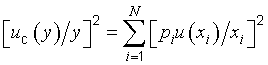(12)

This is of the same form as Equation (11a) but with the combined variance u2c(y) expressed as a relative combined variance [uc(y)y]2 and the estimated variance u2(xi) associated with each input estimate expressed as an estimated relative variance [u(xi)xi]2. [The relative combined standard uncertainty is uc(y)y and the relative standard uncertainty of each input estimate is u(xi)xi, y ≠ 0 and xi ≠ 0.]

NOTE 1   When Y has this form, its transformation to a linear function of variables (see 5.1.5) is readily achieved by setting Xi = Xi,0(1 + δi), for then the following approximate relation results: (Y − Y0)Y0 = ∑Ni=1 piδi. On the other hand, the logarithmic transformation Z = ln Y and Wi = ln Xi leads to an exact linearization in terms of the new variables: Z = ln c + ∑Ni=1 piWi.

NOTE 2   If each pi is either +1 or −1, Equation (12) becomes [uc(y)y]2 = ∑Ni=1[u(xi)xi]2, which shows that, for this special case, the relative combined variance associated with the estimate y is simply equal to the sum of the estimated relative variances associated with the input estimates xi.

## 5.2   Correlated input quantities

5.2.1   Equation (10) and those derived from it such as Equations (11a) and (12) are valid only if the input quantities Xi are independent or uncorrelated (the random variables, not the physical quantities that are assumed to be invariants — see 4.1.1, Note 1). If some of the Xi are significantly correlated, the correlations must be taken into account.

5.2.2   When the input quantities are correlated, the appropriate expression for the combined variance u2c(y) associated with the result of a measurement is(13)
where xi and xj are the estimates of Xi and Xj and u(xixj) = u(xjxi) is the estimated covariance associated with xi and xj. The degree of correlation between xi and xj is characterized by the estimated correlation coefficient (C.3.6)(14)
where r(xixj) = r(xjxi), and −1 ≤ r(xixj) ≤ +1. If the estimates xi and xj are independent, r(xixj) = 0, and a change in one does not imply an expected change in the other. (See C.2.8, C.3.6, and C.3.7 for further discussion.)

In terms of correlation coefficients, which are more readily interpreted than covariances, the covariance term of Equation (13) may be written as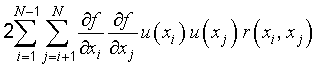(15)

Equation (13) then becomes, with the aid of Equation (11b),(16)

NOTE 1   For the very special case where all of the input estimates are correlated with correlation coefficients r(xixj) = +1, Equation (16) reduces to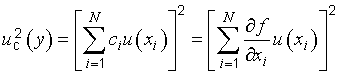The combined standard uncertainty uc(y) is thus simply a linear sum of terms representing the variation of the output estimate y generated by the standard uncertainty of each input estimate xi (see 5.1.3). [This linear sum should not be confused with the general law of error propagation although it has a similar form; standard uncertainties are not errors (see E.3.2).]

EXAMPLE   Ten resistors, each of nominal resistance Ri = 1 000 Ω, are calibrated with a negligible uncertainty of comparison in terms of the same 1 000 Ω standard resistor Rs characterized by a standard uncertainty u(Rs) = 100 mΩ as given in its calibration certificate. The resistors are connected in series with wires having negligible resistance in order to obtain a reference resistance Rref of nominal value 10 kΩ. Thus Rref = f(Ri) = ∑10i=1Ri. Since r(xixj) = r(RiRj) = +1 for each resistor pair (see F.1.2.3, Example 2), the equation of this note applies. Since for each resistor fxi = RrefRi = 1, and u(xi) = u(Ri) = u(Rs) (see F.1.2.3, Example 2), that equation yields for the combined standard uncertainty of Rref, uc(Rref) = ∑10i=1u(Rs) = 10 × (100 mΩ) = 1 Ω. The result uc(Rref) = [∑10i=1u2(Rs)]1/2 = 0,32 Ω obtained from Equation (10) is incorrect because it does not take into account that all of the calibrated values of the ten resistors are correlated.

NOTE 2   The estimated variances u2(xi) and estimated covariances u(xixj) may be considered as the elements of a covariance matrix with elements uij. The diagonal elements uii of the matrix are the variances u2(xi) while the off‑diagonal elements uij(i ≠ j) are the covariances u(xixj) = u(xjxi). If two input estimates are uncorrelated, their associated covariance and the corresponding elements uij and uji of the covariance matrix are 0. If the input estimates are all uncorrelated, all of the off‑diagonal elements are zero and the covariance matrix is diagonal. (See also C.3.5.)

NOTE 3   For the purposes of numerical evaluation, Equation (16) may be written aswhere Zi is given in 5.1.3, Note 2.

NOTE 4   If the Xi of the special form considered in 5.1.6 are correlated, then the terms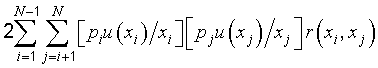must be added to the right‑hand side of Equation (12).

5.2.3   Consider two arithmetic means q and r that estimate the expectations μq and μr of two randomly varying quantities q and r, and let q and r be calculated from n independent pairs of simultaneous observations of q and r made under the same conditions of measurement (see B.2.15). Then the covariance (see C.3.4) of q and r is estimated by(17)
where qk and rk are the individual observations of the quantities q and r and q and r are calculated from the observations according to Equation (3). If in fact the observations are uncorrelated, the calculated covariance is expected to be near 0.

Thus the estimated covariance of two correlated input quantities Xi and Xj that are estimated by the means X‾‾i and X‾‾j determined from independent pairs of repeated simultaneous observations is given by u(xixj) = s(X‾‾iX‾‾j), with s(X‾‾iX‾‾j) calculated according to Equation (17). This application of Equation (17) is a Type A evaluation of covariance. The estimated correlation coefficient of X‾‾i and X‾‾j is obtained from Equation (14)r(xixj) = r(X‾‾iX‾‾j) = s(X‾‾iX‾‾j)[s(X‾‾i)s(X‾‾j)].

NOTE   Examples where it is necessary to use covariances as calculated from Equation (17) are given in H.2 and H.4.

5.2.4   There may be significant correlation between two input quantities if the same measuring instrument, physical measurement standard, or reference datum having a significant standard uncertainty is used in their determination. For example, if a certain thermometer is used to determine a temperature correction required in the estimation of the value of input quantity Xi, and the same thermometer is used to determine a similar temperature correction required in the estimation of input quantity Xj, the two input quantities could be significantly correlated. However, if Xi and Xj in this example are redefined to be the uncorrected quantities and the quantities that define the calibration curve for the thermometer are included as additional input quantities with independent standard uncertainties, the correlation between Xi and Xj is removed. (See F.1.2.3 and F.1.2.4 for further discussion.)

5.2.5   Correlations between input quantities cannot be ignored if present and significant. The associated covariances should be evaluated experimentally if feasible by varying the correlated input quantities (see C.3.6, Note 3), or by using the pool of available information on the correlated variability of the quantities in question (Type B evaluation of covariance). Insight based on experience and general knowledge (see 4.3.1 and 4.3.2) is especially required when estimating the degree of correlation between input quantities arising from the effects of common influences, such as ambient temperature, barometric pressure, and humidity. Fortunately, in many cases, the effects of such influences have negligible interdependence and the affected input quantities can be assumed to be uncorrelated. However, if they cannot be assumed to be uncorrelated, the correlations themselves can be avoided if the common influences are introduced as additional independent input quantities as indicated in 5.2.4.